### Acids, Bases And Salts Class 10th Science Gujarat Board Solution

##### Question 1.What is formed by reaction of non-metal oxide with water ?A. AcidB. BaseC. SaltD. MetalAnswer:Acid is formed by reaction of non-metal oxide with water. This can be illustrated with the help of an example:CO2 + H2O → H2CO3Carbon is a non-metal and thus carbon dioxide is a non-metal oxide. Thus, when a non-metal oxide (CO2) reacts with water, an acid (carbonic acid) is formed.Question 2.Acid + Metal-oxide → ?A. Base + WaterB. Salt + WaterC. Base + SaltD. Metal + SaltAnswer:When a metal reacts with a base, salt and water are produced. The reaction of the acid with metal oxide is similar to the reaction with a base. This is because the metal oxides are basic in nature.Question 3.Which gas is produced by reaction of base with metal ?A. Carbon dioxideB. DioxygenC. DihydrogenD. DinitrogenAnswer:When strong bases react with some amphoteric metals (which can react with both acids and bases), salt and dihydrogen gas are produced.Example: 2NaOH + Zn → Na2ZnO2 + H2Question 4.500 ml aqueous solution is prepared by dissolving 2 moles of HCI in water. What will be the molarity of this solution ?A. 1B. 2C. 3D. 4Answer:Molarity of a solution can be defined as:If one mole of solute is dissolved in One-litre solution, then the concentration of the solution has 1 molarity. So from the above definition, formula for finding molarity can be written as:Molarity = no. of moles ÷ volume of solution in litresFor the given question,No. of moles of HCL = 2Volume in mL = 500mLVolume in litres = 500÷1000 = 0.5LMolarity = 2 ÷ 0.5 = 4MQuestion 5.What is correct for acidic aqueous solution?A. [H3O + ] = 10-7 MB. [H3O + ] > 10-7 MC. [H3O + ] < 10-7 MD. [H3O + ] < [OH-]Answer:Concentration of hydronium ions is equivalent to 10-7M in neutral solutions. Acidic solutions have a greater concentration ofHydronium ions than Hydroxide ions. Thus acidic solutions have hydronium ion concentration greater than 10-7 M.Question 6.Which of the following solutions is the most basic?A. pH = 8.2B. pH = 9.3C. pH = 11.5D. pH = 10.6Answer:As the concentration of hydroxide ions increases, the basicity also increases and the pOH decreases. If pOH decreases that means pH increases. So, In simple words, the basicity increases with increase in pH. Therefore, The solution having pH = 11.5 is most basic.Question 7.Which statement is incorrect ?A. pH scale was presented by S.P.L Sorensen.B. pH scale ranges between 0 to 14.C. pH scale is applicable to only non-aqueous solutions.D. pH scale is applicable to only aqueous solutions.Answer:The pH scale was presented by S.P.L Sorensen to express the concentration of hydronium ions in a solution conveniently. The range of the pH scale is from 0 to 14 and its limitation is that it is applicable to only aqueous solutions. Thus the statement is incorrect.Question 8.How is the exact pH of an aqueous solution measured?A. pH paperB. Litmus paperC. pH meterD. Universal indicatorAnswer:To measure the pH of an aqueous solution approximately, the methods used are: pH paper, litmus paper and universal indicator. All these methods give the general idea of the acidity or basicity of the aqueous solution. The pH meter exactly measures the pH of an aqueous solution and returns a numeric value of pH.Question 9.Which of the following substances is an antacid?A. NaClB. Mg(OH)2C. HClD. H2SO4Answer:As the food enters the stomach, hydrochloric acid is secreted. This decreases the pH in the stomach and becomes helpful in digestion. Sometimes more HCL is secreted to digest certain food products such as eggs, fish etc. This causes the pain in our stomachs and termed as acidity. Basic substances neutralize this acid and reduce the pain. They are known as antacids.Question 10.The aqueous solution having pH 11 is how many times less basic than aqueous solution having pH 8?A. 3B. 30C. 300D. 1000Answer:A) solution with pH = 11pH + pOH = 14Therefore, pOH = 14-11 = 3Hence, [OH-] = 10-3B) solution with pH = 8pOH = 14-8 = 6Hence, [OH-] = 10-6So the solution with pH = 11 is (10-3÷10-6) 1000 times more basic than the solution with pH = 8Question 11.Which of the following is strong acid?A. Acetic acidB. Citric acidC. Nitric acidD. Oxalic acidAnswer:Nitric acid completes dissociates in aqueous solution. So it releases more H3O +. Thus it is a strong acid.Reaction: HNO3 + H2O → H3O + + NO3-If 1M Nitric acid is taken then it will completely dissociate in aqueous solution to give 1M H3O +.Acetic acid, oxalic acid, and citric acid do not dissociate completelyin aqueous solution, thus they are weak acids.Question 12.What type of substance is NH3A. Strong acidB. Weak acidC. Strong baseD. Weak baseAnswer:NH3 acts as a base because it accepts H + when dissolved in the water. Ammonia is known as a weak base because its hydroxide (NH4OH) does not dissociate completely in its aqueous solution. Thus, it is a weak base.Question 13.pH + pOH = ?A. 7B. 0C. 14D. 10Answer:pH = -log10[H + ]pOH = -log10[OH-]Since the distilled water is neutral,[H + ] = [OH-] = 10-7[H + ] × [OH-] = 10-7 × 10-7[H + ] × [OH-] = 10-14Taking negative logarithm on both sides,-log10[H + - log10[OH-] = -log10[10-14]pH + pOH = 14Question 14.Which formula is correct?A. Mole = Molecular mass / WeightB. Mole = Weight / Molecular massC. Mole = Weight / LitreD. Mole = Molecular mass / LitreAnswer:Unit of molecular mass is gram per mole. It means that molecular mass is the mass of one mole. Weight is the mass of some unknown no. of moles.So by dividing the given weight by molecular mass, we can find the unknown no. of moles.Question 15.What will be the pH of aqueous solution of NH4 Cl ?A. pH = 7B. pH > 7C. pH < 7D. pH = 0Answer:NH4 Cl is a salt produced by the neutralization reaction between a strong acid (HCl) and a weak base (NH4OH). The reaction between them can be represented as follows:HCL + NH4OH → NH4CL + H2OHydrolyses of NH4CL tales place as follows:NH4+ + H2O → NH3 + H3O +Its produces H3O + and that’s why the aqueous solutions of salt produced by neutralization of strong acid and a weak base are acidic in nature. Therefore, pH < 7.Question 16.Which of the following solutions will have pH = 2?A. 0.01 M HClB. 0.02 M HClC. 0,01M H2SO4D. 0.02M H2SO4Answer:The formula to calculate pH is as follows:pH = -log10[H + ]In this question we are already provided with pH, so we have to calculate the concentration of [H + ] from the above formula.2 = -log10[H + ]So, the [H + ] = 10-2 from the above equation. Therefore, 0.01M HClwill have a pH of 2.Question 17.What will be OH- concentration in an aqueous solution having pH = 8?A. 1 x 10-8 MB. 1 x 10-6 MC. 8 x 10-6 MD. 8 x 10-8 MAnswer:The formula to calculate pOH is as follows:pOH = -log10[OH-]Also, the relation between pH and pOH is: pH + pOH = 14Therefore, pOH = 14- 8 = 6Now, using the formula of pOH:6 = -log10[OH-]Therefore, the concentration of hydroxide ions is 1 x 10-6 M.Question 18.If the pH of aqueous solutions A, B, C and D are 1.9, 2.5, 2.1 and 3.0 then what will be the order of acidity ?A. A < C < B < DB. D < C < B < AC. D < B < C < AD. D > C > B > AAnswer:As the concentration of H + ions increase, the acidity increases, and the pH decreases. Thus, we can say that lesser the pH more will be the acidity. Therefore, solution A will have maximum acidity as it has the least pH followed by solution C, followed by solution B and solution D is least acidic as it has a maximum pH of 3.The given picture explains the concept: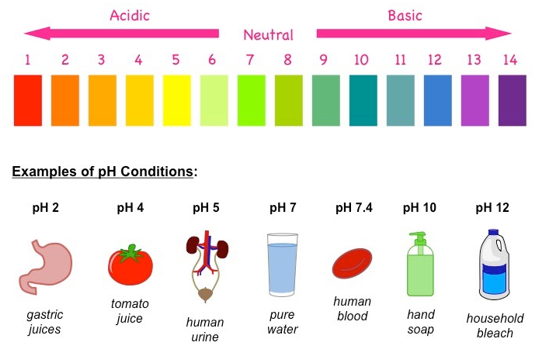Question 19.Which solution will be basic ?A. [H3O + ] = 10-5MB. [H3O + ] = 10-12MC. [H3O + ] = 10-7MD. [H3O + ] = 10-4MAnswer:Concentration of hydronium ions is equivalent to 10-7 M in neutral solutions. For basic solutions the concentration of [H3O + ] is less than 10-7 M. In the above options, only option B has [H3O + ] less than 10-7 M. Thus, option B will be basic.Question 20.Which substance is present in poison of honey bee?A. LimeB. Calcium phosphateC. MelittinD. Pepsin.Answer:When the honey bee bites, we feel the pain and irritation because of the presence of acid Melittin in the poison of the honey bee. It contains 26 amino acids. To get relief from the pain, aqueous solutions of basic substances are applied to neutralize the acid.Question 21.Write names of two Arrhenius acids and bases.Answer:Arrhenius acid is a hydrogen-containing substance which produces hydrogen ion (H + ) in its aqueous solution.Examples of two such acids are Nitric acid and hydrochloric acid.HNO3→ H + + NO3-HCl → H + + Cl-Arrhenius base is hydroxide containing substance and which produces OH- ion in its aqueous solution.Examples of two such bases are sodium Hydroxide and potassium hydroxide.NaOH → Na + + OH-KOH → K + + OH-Question 22.Write products of reactions of acid with metal.Answer:On the reaction of the acid with metal, the products formed aredihydrogen gas and salt corresponding to the metal.Acid + Metal → salt of metal + dihydrogen gasFor example: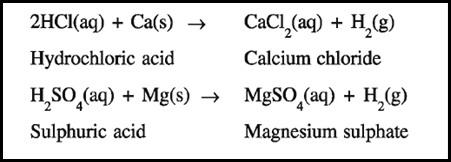Question 23.Mention names of four methods of expressing the concentration of a solution.Answer:The amount of solute in relation to the amount of solvent iscalled concentration. The concentration of a solution can be expressed in different ways. Four methods of expressing the concentration of a solution are as follows:•Molarity•Normality•Molality•Percentage proportionMolarity and normality are more commonly used.Question 24.Which scientist presented the pH scale? Write the formula of pH.Answer:The pH scale was presented by S.P.L Sorensen to express the concentration of hydronium ions in a solution conveniently. The range of the pH scale is from 0 to 14 and it is applicable to only aqueous solutions.The formula to calculate pH is: pH = -log10[H3O + ]Question 25.Write names of two methods to measure approximate pH of aqueous solution.Answer:Approximate methods to measure pH are those methods which give the general idea about the basicity and acidity of a given solution.Two such methods are:1. Litmus paper: if the colour of litmus paper changes from blue to red it indicates the acidic nature and if the colour of litmus paper changes from red to blue it indicates basic nature. Thus it is an approximate method.2. pH paper: pH paper is dipped in the solution whose pH is to be determined. There occurs a colour change. By observing the colour of the pH paper, we can get a general idea of the pH of the solution.Question 26.Mention names of two strong acids and two strong bases.Answer:Strong acids are those acids which completely dissociate in their aqueous solutions.Examples:•Hydrochloric acid•Nitric acidStrong bases are those bases which completely dissociate in their aqueous solutions.Examples:•Sodium hydroxide•Potassium hydroxide.Question 27.Write names of two weak acids and two weak bases.Answer:Weak acids are those acids which partially dissociate in their aqueous solutions.Examples:•Oxalic acid•Citric acidWeak bases are those bases which partially dissociate in their aqueous solutions.Examples:•Ammonium hydroxide•AmmoniaQuestion 28.Give definitions:i. Arrhenius acidii. Arrhenius baseiii. Bronsted-Lowry acidiv. Bronsted-Lowry basev. Concentration of solutionvi. pH of solutionvii. pOH of solutionviii. Strong acidix. Weak acidx. Strong basexi. Weak basexii. Neutralisation reactionxiii. 1M concentrationAnswer:i. Arrhenius acid is a hydrogen-containing substance which produces hydrogen ion (H + ) in its aqueous solution.Examples of such acids are Nitric acid and hydrochloric acid.HNO3→ H + + NO3-HCl → H + + CL-Both contain Hydrogenand produce H +ii. Arrhenius base is hydroxide containing substance and which produces OH- ion in its aqueous solution.Examples of two such bases are sodium Hydroxide and potassium hydroxide.NaOH → Na + + OH-KOH → K + + OH-Both contain Hydroxide and produces OH-iii. The substances which donate protons [H + ] to the other substance are called bronsted-lowry acids. The species responsible for acidity is not [H + ] but [H3O + ].Examples of bronsted-lowry acids are: HCl, HNO3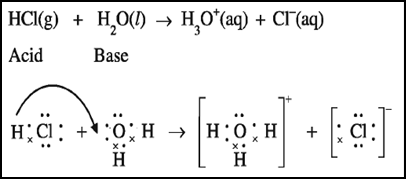iv. Bronsted-lowry base is a substance which accepts proton from other substance. For example ammonia.When ammonia is dissolved in the water, it accepts a proton from water and thus behaves as a bronsted-lowry base.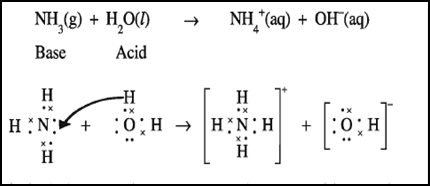v. A system resulting when a solute is dissolved in a solvent is called a solution. The substance which is present in lesser proportion is called solute and the substance which is in present in a larger proportion is known as a solvent.The amount of solute in relation to the amount of solvent iscalled concentration. The concentration of a solution can be expressed in terms of molarity, normality etc.vi. The pH is defined as the negative logarithm to the base 10 of the concentration of H3O + in aqueous solution. In simple words, pH is used to express the hydronium ion concentration in a solution.Less the pH more will the hydronium ion concentration.pH = -log10[H3O + ]vii. The pOH is defined as the negative logarithm to the base 10 of the concentration of hydroxide ions in the aqueous solution. In simple words, pOH is used to express the hydroxide ion concentration in a solution. Less the pOH more will be hydroxide ion concentration.pOH = -log10[OH-]viii. Strong acids are those acids which completely ionize or dissociate in their aqueous solutions.Examples:•Hydrochloric acid•Nitric acidDissociation of nitric acid: HNO3→ H + + NO3-Dissociation of hydrochloric acid: HCl → H + + CL-ix. Weak acids are those acids which partially ionize or dissociate in their aqueous solutions.Examples:•Oxalic acid•Citric acidx. Strong bases are those bases which completely ionise or dissociate in their aqueous solutions.Examples:•Sodium hydroxide•Potassium hydroxide.Dissociation of Sodium hydroxide: NaOH → Na + + OH-Dissociation of potassium hydroxide: KOH → K + + OH-xi. Weak bases are those bases which partially ionize or dissociate in their aqueous solutions.Examples:•Ammonium hydroxide•Ammoniaxii. When a reaction takes place between an acid and a base, salt and water are produced. This reaction is called a neutralisation reaction. In this reaction acid and base mutually nullify each other’s properties. An example of such a reaction is: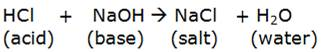xiii. The amount of solute in relation to the amount of solvent iscalled concentration. The concentration of a solution can be expressed in terms of molarity, normality etc. 1M concentration can be defined as 1 mole of a substance dissolved in 1 litre of a solution.Question 29.Mention the formula, name and physical state of the products of following reactions:1. CO2(g) + H2O(l)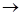2. Li2O(s) + H2O(l)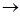3. H2SO4(aq) + 2KOH(aq)4. 2HCL(aq) + Ca(s)5 2HNO3(aq) + CaO(s)6. 2HCL(aq) + Na2CO3(aq)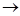7. NaOH(aq) + HCl(aq)8. BaCl2(aq) + H2SO4(aq)9. Ca(OH)2(aq) + CO2(g)10. HNO3(aq) + NH4OH(aq)11. Na2SO4(aq) + Ba(OH)2(aq)12. AgNO3(aq) + NaCl(aq)Answer:1. CO2(g) + H2O(l)H2CO3 (aq)(carbonic acid)2. Li2O(s) + H2O(l)2LiOH (aq)(Lithium hydroxide)3. H2SO4(aq) + 2KOH(aq)K2SO4 (aq) + 2H20(l)(potassium (water) Sulphate)4. 2HCL(aq) + Ca(s)CaCl2(aq) + H2(g)(calcium chloride) (dihydrogen gas)5 2HNO3(aq) + CaO(s)Ca(NO3)2 (aq) + H2O(l)(calcium nitrate) (water)6. 2HCL(aq) + Na2CO3(aq)2NaCl(aq) + H2O(l) + CO2(g)(sodium chloride) (water) (carbon Dioxide)7. NaOH(aq) + HCl(aq)NaCl(aq) + H2O(l)(sodium chloride) (water)8. BaCl2(aq) + H2SO4(aq)BaSO4(s) + HCl(aq)(barium sulphate) (hydrochloric acid)9. Ca(OH)2(aq) + CO2(g)CaCO3(s) + H2O(l)(calcium carbonate) (water)10. HNO3(aq) + NH4OH(aq)NH4NO3(aq) + H2O(l)(ammonium nitrate) (water)11. Na2SO4(aq) + Ba(OH)2(aq)BaSO4 (s) + 2NaOH(aq)(barium sulphate) (sodium Hydroxide)12. AgNO3(aq) + NaCl(aq)AgCl(s) + NaNO3(aq)(silver chloride) (sodium nitrate)Question 30.Answer the following questions:1. Write two chemical properties of an acid.2. Write two chemical properties of the base.3. Write two chemical properties of salt.4. Deduce pH + pOH = 145. Explain the importance of pH in the digestion of food.6. Write chemical equations for four neutralisation reactions.Answer:1. Two properties of acids are are as follows:•The reaction of the acid with metal:Acids on reaction with metals produce dihydrogen gas and salt corresponding to the metal.Acid + metal → salt + dihydrogen gas•The reaction of acid with base:Salt and water are formed when acids react with bases. This reaction is called a neutralisation reaction.Acid + Base → salt + water2. two properties of bases are as follows:•The reaction of the base with acid:Salt and water are formed when acids react with bases. This reaction is called a neutralization reaction.Acid + Base → salt + water•The reaction of the base with non-metal oxideThis reaction is also similar to the reaction of base with acids. Salt and water are produced in this reaction also.Base + Non-metal oxide → salt + water3. two properties of salts are as follows:•Salt reacts with other acids to form another salt and acidSalt + acid → other salt + acid•Salt reacts with another base to form other salt and base.Salt + base → other salt + base4. pH = -log10[H + ]pOH = -log10[OH-]Since the distilled water is neutral,[H + ] = [OH-] = 10-7[H + ] × [OH-] = 10-7 × 10-7[H + ] × [OH-] = 10-14Taking negative logarithm on both sides,-log10[H + ] - log10[OH-] = -log10[10-14]pH + pOH = 145. As the food enters the stomach, hydrochloric acid is secreted. This decreases the pH in the stomach. The pH in the stomach turns between 1 and 3. In the decreased pH, the enzyme pepsin becomes active and helps in the process of digestion.6. When a reaction takes place between an acid and a base, salt and water are produced. This reaction is called a neutralisation reaction. In this reaction acid and base mutually nullify each other’s properties.Four such reactions are as follows:•HCl + NaOH → NaCl + H2O•CH3COOH + NH4OH → CH3COONH4 + H2O•HNO3 + KOH → KNO3 + H2O•H2SO4 + 2KOH → K2SO4 + 2H2OQuestion 31.Solve the following problems:1. Calculate pH of an aqueous solution having H3O + concentration equal to 7.9 x 10-11 M. Which nature, acidic basic or neutral will be possessed by this aqueous solution.2. Calculate pH of 0.00424 M aqueous solution of KOH.3. How many times concentrated will be the aqueous solution having pH 11.9 as compared to aqueous solution having pH 8?4. How will you prepare 500ml aqueous solution of 0.2 M H2SO4?5. How will you prepare 125 ml 0.03 M aqueous solution of KOH.Answer:1. [H3O + ] = 7.9 x 10-11 MThe pH can be calculated using the formula:pH = -log10[H3O + ]putting the value of hydronium ion concentration in above equation, we get:pH = -log10[7.9 x 10-11]pH = 11 - log10[7.9]using the log table, we get:pH = 11-0.8976 = 10.10Since the pH of the solution comes out to be greater than 7, thus the solution is basic in nature.2. [OH-] = 0.00424 MThe formula to calculate pOH is:pOH = -log10[OH-]Putting the value of hydroxide ion concentration in above equation:pOH = -log10[0.00424]= -log10[4.24 × 10-3]= 3 - log10[4.24]= 3 – 0.6274= 2.37Using the relation, pH + pOH = 14pH = 14- 2.37 = 11.633. A) for solution of pH = 11.9, pOH = 2.1We know that, pOH = -log10[OH-]Putting given pOH value in above equation we get:2.1 = -log10[OH-]log10[OH-] = -2.1log10[OH-] = 3 – 2.1 – 3log10[OH-] = -3 + 0.9[OH-] = antilog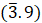= 7.943 × 10-3 MB) solution with pH = 8, pOH = 66 = - log10[OH-][OH-] = 10-6Dividing the hydroxide ion concentration of s by Ist solution by second solution, we get: (7.943 × 10-3 ÷ 10-6 ) = 7943 times.4. the formula used to find molarity is: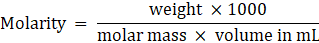Putting the value of molarity = 0.2 MVolume in mL = 500 mLMolar mass = 98 g/mol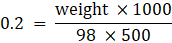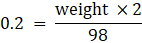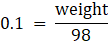Weight = 9.8 g.So, by adding 9.8 g of H2SO4 in the 500mL water, we can prepare its 0.2M solution.5. the formula used to find molarity is:Molarity = 0.03 MVolume in mL = 125 mLMolar mass of KOH = 56g/ mol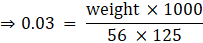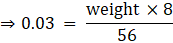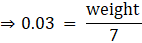Weight = 0.21 g.So by adding 0.21 g of KOH in 125 mL water, its 0.03 solution can be prepared.Question 32.Answer the following questions in detail :1. Explain giving example, Arrhenius acid-base theory, Mention the limitations of this theory.2. Discuss Bronsted-Lowry Acid-base theory.3. Discuss methods to measure the pH of the aqueous solution.Answer:1. Arrhenius acid is a hydrogen-containing substance which produces hydrogen ion (H + ) in its aqueous solution.Examples of such acids are Nitric acid and hydrochloric acid.HNO3→ H + + NO3-HCl → H + + CL-Both contain Hydrogenand produce H +Arrhenius base is hydroxide containing substance and which produces OH- ion in its aqueous solution.Examples of two such bases are sodium Hydroxide and potassium hydroxide.NaOH → Na + + OH-KOH → K + + OH-Both contain Hydroxide and produces OH-Limitations of this theory are as follows:•It is applicable to aqueous solutions only.•It could not explain the basicity of ammonia as ammonia doesn’t contain hydroxide ion.•It said that H + is responsible for the acidity while H + is very unstable and readily combines with water to form hydronium ion.2. The substances which donate protons [H + ] to the other substance are called bronsted-lowry acids. The species responsible for acidity is not [H + ] but [H3O + ].Examples of bronsted-lowry acids are: HCl, HNO3The bronsted-lowry base is a substance which accepts a proton from other substance. For example ammonia.When ammonia is dissolved in the water, it accepts a proton from water and thus behaves as a bronsted-lowry base.3. There are two types of methods to measure the pH of a solution. Approximate methods are used to get the general idea about the acidity and basicity of a given solution and exact method is used to know the exact numeric value of pH.Approximate methods:1. Litmus paper: if the colour of litmus paper changes from blue to red it indicates the acidic nature and if the colour of litmus paper changes from red to blue it indicates basic nature. Thus, it is an approximate method.2. pH paper: pH paper is dipped in the solution whose pH is to be determined. There occurs a colour change. By observing the colour of the pH paper, we can get a general idea of the pH of the solution.Exact method:1. pH-meter: pH-meter is an instrument used for exact pH value measurement. The pH-meter has two electrodes which are dipped in the solution whose pH is to be determined. The electrodes are attached through a circuit to an indicating box where the pH of the solution is read.Question 33.Solve the following problems :1. [OH-] in aqueous solution A is = 4.3 x10-4M and [H3O + ] in aqueous solution of B is 7.3 x 10-10 M, pH of which solution will be less? Which solution will be more basic?2. Calculate the concentration of OH- in aqueous solution having pH value 9.3.3. 8 gram NaOH is dissolved in water and the aqueous solution is made to 5 litres. Find pH of this solution.Answer:1. In distilled water: [H3O + ] × [OH-] = 10-14For solution A, [H3O + ] = 10-14 ÷ [OH-]= 10-14 ÷ (4.3 × 10-4)= 2.32 × 10-11 MFor solution B, [H3O + ] = 7.3 × 10-10 M•Since the hydronium ion concentration of solution B is more, so its pH will be less.•The solution having the more pH will be more basic. As solution A will have more pH so it will be more basic.2. using the relation, pOH + pH = 14pOH = 14 – pH= 14 – 9.3= 4.7Using, pOH = -log10[OH-]4.7 = -log10[OH-]log10[OH-] = -4.7log10[OH-] = 5 -4.7 -5log10[OH-] = -5 + 0.3log10[OH-] =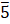+ 0.3[OH-] = antilog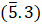[OH-] = 1.99 × 10-53. molar mass of NaOH = atomic mass of Na + Atomic mass of O + atomic mass of H = 23 + 16 + 1 = 40g/ mol.Volume of solution = 5 litresGiven weight = 8gNo. of moles = given weight ÷ molar mass= 8g ÷ 40 g/mol= 0.2 moleMolarity = no. of moles ÷ volume in litres= 0.2 ÷ 5= 0.04 MTherefore, the hydroxide ion concentration [OH-] = 0.04 MpOH = -log10[OH-]pOH = -log10[ 4 × 10-2]pOH = 2 -log10[ 4]pOH = 2- 0.6020 = 1.39using, pOH + pH = 14pH = 14 – pOHpH = 14 - 1.39 = 12.61Question 34.Answer the following question in detail:1. With reference to PH scale,I. i.Write formula of pH and pOHii Mention pH and concentration of H3O + or OH- in acidic, basic and neutral aqueous solutions.iii Mention limitations of pH scale.2. Explain the importance of pH in everyday life.3. “The aqueous solution of the salt produced by neutralization of weak acid and strong base possesses basic nature, while an aqueous solution of salt produced by neutralization of a weak base and strong acid possesses acidic nature” – Explain.Answer:1 i.pOH = -log10[OH-]pH = -log10[H3O + ]ii. 1.acidic solutions have hydronium ion concentration greater than 10-7 M and hydroxide ion has a concentration of less than 10-7 M. Thus the pH of the acidic solution is less than 7.2. Basic solutions have hydroxide ion concentration greater than 10-7 M and hydronium ion has a concentration of less than 10-7 M. Thus the pH is greater than 7.3. in a neutral solution, the concentration of hydronium ions is equal to that of hydroxide ions which is equal to 10-7 M. thus the pH is 7.iii. The limitations of the pH scale are as follows:•pH scale is applicable to aqueous solutions only.•pH scale is valid for aqueous solutions having concentration bless than 1 M because its range is 0 to 14.2. the importance of pH in our daily life is as follows:•Importance of pH for the existence of living beings:The various reactions that occur inside living beings require a specific pH range of 7.0 to 7.8. the living beings are not able to survive large changes in pH.•Importance of pH in digestive systems:As the food enters the stomach, hydrochloric acid is secreted. This decreases the pH in the stomach. The pH in the stomach turns between 1 and 3. In the decreased pH, the enzyme pepsin becomes active and helps in the process of digestion.•Importance of pH in stopping the decay of teeth:When the pH on the inner side of our mouth is less than 5.5 then the tooth decay starts. To prevent the teeth from decaying we use basic toothpastes which neutralise the acid produced inside our mouth.3. The aqueous solution of the salt produced by neutralization of weak acid and strong base possesses basic nature. This is because the salt produced undergoes hydrolyzes during which it combines with H + ions from water liberating free hydroxide ions.For example: Sodium acetate is a salt made by the reaction between strong base NaOH and weak acetic acid.It undergoes hydrolyzes as follows to liberate hydroxide ions: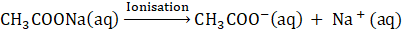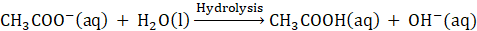An aqueous solution of salt produced by neutralization of a weak base and strong acid possesses acidic nature. This is because such salt undergoes hydrolyzes and produces hydronium ions.For example ammonium nitrate is made from the reaction between strong acid nitric acid and weak base ammonium hydroxide.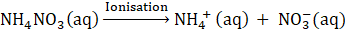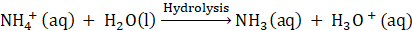Question 35.Solve the following problems:1. pH of an aqueous solution of potassium hydroxide at 298 K temperature is 11.65. The initial volume of this solution is made 6 times by addition of water. What will be the pH of the diluted solution ?2. What will be the change in the value of pH if the concentration of the aqueous solution of HNO3is increased to 0.05 M from 0.03 M ?Answer:1. pH = 11.65Therefore, pOH = 14 – 11.65 = 2.35Using, pOH = -log10[OH-]Putting the value of pOH in above equation:2.35 = -log10[OH-]log10[OH-] = -2.355log10[OH-] = 3 -2.35 -3log10[OH-] = -3 + 0.65log10[OH-] =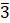+ 0.65[OH-] = antilog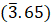[OH-] = 4.46 × 10-3Now if volume is made 6 times then the concentration will decrease 6 times. So the new concentration is = 4.46× 10-3÷ 6= 7.44 × 10-4So new pOH = -log10[OH-]= -log10[7.44 × 10-4]= 4-log10[7.44]= 4- 0.871= 3.129Therefore, new pH is 14 – 3.129 = 10.8712. A) when concentration is 0.03 MUsing, pH = -log10[H3O + ]= -log10[0.03]= -log10[3 × 10-2]= 2 -log10= 2 – 0.477= 1.52B) When concentration is 0.05 MUsing, pH = -log10[H3O + ]= -log10[0.05]= -log10[5 × 10-2]= 2 -log10= 2 – 0.699= 1.30So the change in pH is = 1.52 – 1.30= 0.22

PDF FILE TO YOUR EMAIL IMMEDIATELY PURCHASE NOTES & PAPER SOLUTION. @ Rs. 50/- each (GST extra)

HINDI ENTIRE PAPER SOLUTION

MARATHI PAPER SOLUTION

SSC MATHS I PAPER SOLUTION

SSC MATHS II PAPER SOLUTION

SSC SCIENCE I PAPER SOLUTION

SSC SCIENCE II PAPER SOLUTION

SSC ENGLISH PAPER SOLUTION

SSC & HSC ENGLISH WRITING SKILL

HSC ACCOUNTS NOTES

HSC OCM NOTES

HSC ECONOMICS NOTES

HSC SECRETARIAL PRACTICE NOTES

2019 Board Paper Solution

HSC ENGLISH SET A 2019 21st February, 2019

HSC ENGLISH SET B 2019 21st February, 2019

HSC ENGLISH SET C 2019 21st February, 2019

HSC ENGLISH SET D 2019 21st February, 2019

SECRETARIAL PRACTICE (S.P) 2019 25th February, 2019

HSC XII PHYSICS 2019 25th February, 2019

CHEMISTRY XII HSC SOLUTION 27th, February, 2019

OCM PAPER SOLUTION 2019 27th, February, 2019

HSC MATHS PAPER SOLUTION COMMERCE, 2nd March, 2019

HSC MATHS PAPER SOLUTION SCIENCE 2nd, March, 2019

SSC ENGLISH STD 10 5TH MARCH, 2019.

HSC XII ACCOUNTS 2019 6th March, 2019

HSC XII BIOLOGY 2019 6TH March, 2019

HSC XII ECONOMICS 9Th March 2019

SSC Maths I March 2019 Solution 10th Standard11th, March, 2019

SSC MATHS II MARCH 2019 SOLUTION 10TH STD.13th March, 2019

SSC SCIENCE I MARCH 2019 SOLUTION 10TH STD. 15th March, 2019.

SSC SCIENCE II MARCH 2019 SOLUTION 10TH STD. 18th March, 2019.

SSC SOCIAL SCIENCE I MARCH 2019 SOLUTION20th March, 2019

SSC SOCIAL SCIENCE II MARCH 2019 SOLUTION, 22nd March, 2019

XII CBSE - BOARD - MARCH - 2019 ENGLISH - QP + SOLUTIONS, 2nd March, 2019

HSC Maharashtra Board Papers 2020

(Std 12th English Medium)

HSC ECONOMICS MARCH 2020

HSC OCM MARCH 2020

HSC ACCOUNTS MARCH 2020

HSC S.P. MARCH 2020

HSC ENGLISH MARCH 2020

HSC HINDI MARCH 2020

HSC MARATHI MARCH 2020

HSC MATHS MARCH 2020

SSC Maharashtra Board Papers 2020

(Std 10th English Medium)

English MARCH 2020

HindI MARCH 2020

Hindi (Composite) MARCH 2020

Marathi MARCH 2020

Mathematics (Paper 1) MARCH 2020

Mathematics (Paper 2) MARCH 2020

Sanskrit MARCH 2020

Sanskrit (Composite) MARCH 2020

Science (Paper 1) MARCH 2020

Science (Paper 2)

MUST REMEMBER THINGS on the day of Exam

Are you prepared? for English Grammar in Board Exam.

Paper Presentation In Board Exam

How to Score Good Marks in SSC Board Exams

Tips To Score More Than 90% Marks In 12th Board Exam

How to write English exams?

How to prepare for board exam when less time is left

How to memorise what you learn for board exam

No. 1 Simple Hack, you can try out, in preparing for Board Exam

How to Study for CBSE Class 10 Board Exams Subject Wise Tips?

JEE Main 2020 Registration Process – Exam Pattern & Important Dates

NEET UG 2020 Registration Process Exam Pattern & Important Dates

How can One Prepare for two Competitive Exams at the same time?

8 Proven Tips to Handle Anxiety before Exams!